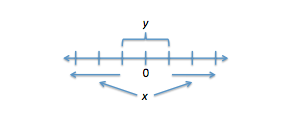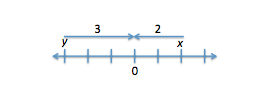• Take advantage of our featured Black Friday/Cyber Monday deals

• Take advantage of our featured Black Friday/Cyber Monday deals

# Breaking Down a GMATPrep Absolute Value Problem

by , Dec 8, 2010A student taking the test soon has requested an article on Absolute Value, so thats what were going to tackle this week; the problem is from GMATPrep.

Is |x|>|y|?

(1) (x^2) > (y^2)

(2) x > y

The first thing youll probably notice: I didnt include the answer choices. The five Data Sufficiency answer choices are always the same, so we should have those memorized. If you dont have them memorized yet, add this to your to do list.

Just in case, here are the five choices (in casual language, not official language):

(A) statement 1 works but statement 2 does not work

(B) statement 2 works but statement 1 does not work

(C) the statements do NOT work alone, but they DO work together

(D) each statement works by itself

(E) nothing works, not even using them together

Okay, now that weve got that out of the way, lets tackle this problem! This ones a tricky theory question; theyre asking us about the concept of absolute value (as opposed to asking us to do more straightforward calculations with absolute value).

I have two variables, x and y, and Im asked a yes/no question about a particular inequality. My task is to determine whether I can answer this question always yes, always no, or sometimes yes, sometimes no given various pieces of information in the statements. An always yes or always no answer is sufficient to answer the question. A sometimes yes, sometimes no answer is NOT sufficient to answer the question.

First, I want to decide whether I can figure anything out just from the question stem (before I start addressing the statements). In this case, the question stem asks about a single inequality and provides absolute value symbols in that inequality. The absolute value of a particular number refers to how far that number is from zero on the number line without regard to the sign (positive or negative) of that number. For example, the absolute value of -5 is 5, because -5 is five units away from zero on the number line.

The question is asking whether the absolute value of x is greater than the absolute value of y. Sketch a quick number line. Draw a few tick marks and label the one in the middle zero (we want some positive and some negative, since this is an absolute value problem). Now think. What would have to be true in order for that statement to be true?

Pick a value for y and place it on your number line; show where y would be if it were either positive or negative (but the same number / distance from zero). Where would x have to go in order to make the statement true?

Your drawing might look something like this:What does the drawing mean in words? In order for the question stem to be true, the distance from zero to x would have to be greater than the distance from zero to y, regardless of the sign. We can simplify that statement a little. The distance from zero to x can be expressed as the magnitude of x. So heres the question in normal language:

Is the magnitude of x greater than the magnitude of y?

Next, I glance at the statements to decide which one I want to address first. If I think one is noticeably easier than the other, though, then I start with the easier one; otherwise, I start with statement 1. Do you think one statement is easier on this problem?

I think statement 2 is easier so were going to start with statement 2. (Note: determining the relative difficulty of the two statements is a somewhat subjective call; different people will disagree and thats okay. Start with the one you think is easier.)

(2) x > y

I have two choices here: I can try some numbers or I can think this through theoretically (if I think I know the theory well enough). Well discuss both ways.

What kinds of number combinations could make this true? For example, x could be 3 and y could be 2. That would make the statement x > y valid, so Im allowed to try those numbers. If x = 3 and y = 2, is the answer to my question yes, no, or maybe? (Look at your number line.) The answer is yes.

Now that I have found one example where I can answer yes to my question, my next goal is to see whether I can think of an example that would give me a no answer.What Im really trying to do here is figure out whether theres a pair of numbers that will make the expression x > y true but for which the magnitude of x is not actually greater than the magnitude of y. What about one positive and one negative? Try x = 2 and y = -3. The expression x > y true, but the magnitude of x is now less than the magnitude of y. The answer to our question is no this time.Alternatively, I can think through the above theoretically. This is often faster than trying numbers, but it is also harder: if I dont know the theory well enough, I either wont be able to tackle the statement this way or Ill be more likely to make a mistake with it. If I do know the theory here, then I also know that placing x > y on a number line is simply a matter of placing x somewhere to the right of y. The question, though, is asking about the distance from x or y to zero. I can place x and y anywhere, so long as x is to the right of y, and the possibilities include placing y closer to zero or placing x closer to zero.

A yes and no answer is insufficient. Eliminate choices B and D.

Now we have to tackle the more annoying (to me!) statement: 1.

[pmath]x^2[/pmath] > [pmath]y^2[/pmath]

Many people will first think: if plain old x < y didnt work, is this one really going to work? But you know the GMAT loves to trap us; let's get to work!

Again, we can try numbers or think things through theoretically. Lets try numbers first and, wherever it makes sense, try to reuse the numbers you used for the first statement you evaluated (because then you can reuse some of your work!). We first tried x = 3 and y = 2; do those numbers make this statement true? Yes: 9 > 4. Is the answer to our question yes or no using these numbers? Yes, the magnitude of x is greater than the magnitude of y.

How about our no numbers from the 2nd statement? Can we also use those for this 1st statement? We tried x = 2 and y = -3. Hmm. Plugging those in for the expression in statement 1 yields 4 > 9. Thats not true, so we cant use these numbers. Are there other numbers that do make the expression [pmath]x^2[/pmath] > [pmath]y^2[/pmath]true and that also yield a no answer to our question?

Heres where trying numbers gets tricky. It turns out that we could sit here all day but wed never find numbers that make the expression [pmath]x^2[/pmath] > [pmath]y^2[/pmath]true and that also yield a no answer to our question because this statement is sufficient to answer the question yes always. On the test, youd try three or four sets of different numbers (with different characteristics positives and negatives, fractions, etc.) and, if you kept getting a yes answer, youd go with it, though you wouldnt be 100% positive that you were right (you could have just failed to test the right combination of numbers).

In this case, youd either try a few different sets and then go with the consistent answer youre getting or you would see whether you could think it through theoretically. So lets test the theory now.

What characteristics would the numbers, x and y, have to have in order to make the expression [pmath]x^2[/pmath]>[pmath]y^2[/pmath]true? The signs wouldnt matter. What would matter is only the basic number, stripped of its sign. (Thats interesting just like the signs dont matter in absolute value) What would have to be true of the basic numbers (ignoring the signs)? Hmm. The basic number for x would have to be larger than the basic number for y. Whats the basic number when disregarding the sign? Hey thats just the magnitude or the absolute value of the number! So, in fact, statement 1 is literally defining the expression |x|>|y|. Excellent! Statement 1 is sufficient.

Note: The above is true even for fractions between zero and one. When you square a fraction between zero and one, that number gets smaller instead of getting larger, but when you square a smaller fraction and a larger fraction, the square of the smaller will still be smaller than the square of the larger. For example, if x = 1/2 and y = 1/3, the square of x is 1/4 and the square of y is 1/9. The x value is larger than the y value and the [pmath]x^2[/pmath]value is larger than the [pmath]y^2[/pmath]value.

The correct answer is A. Also, in future, I want to remember that the expression [pmath]x^2[/pmath]>[pmath]y^2[/pmath]can be rephrased as |x|>|y|. Add this one to your list of Unscramble the Code rephrasings.

## Key Takeaways for Solving Theoretical Absolute Value Problems:

(1) Its worth taking the time to manipulate or simplify the expressions, equations, or inequalities using absolute value symbols. On theory problems, sketch out a number line and use that to understand what the text is really saying or asking. Know how to solve absolute value equations or inequalities algebraically as well (we didnt have a problem that required this today).

(2) If you know the theory inside and out, think it through theoretically; if not, test some numbers. Either way, on data sufficiency yes/no questions, your goal is to try to find a yes and a no. If you can, then you know the info was not sufficient; if you keep getting only yes answers or only no answers, then its probably the case that the info is sufficient.

* GMATPrep questions courtesy of the Graduate Management Admissions Council. Usage of this question does not imply endorsement by GMAC.